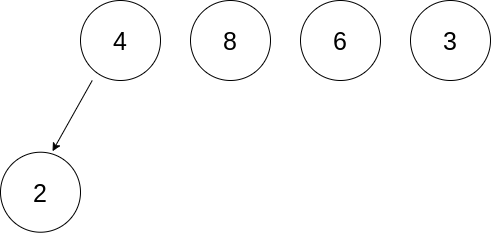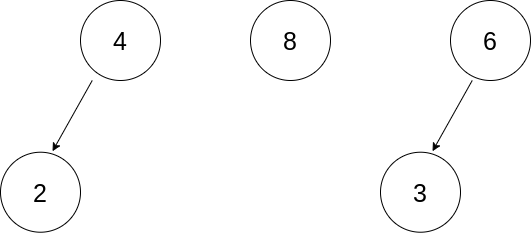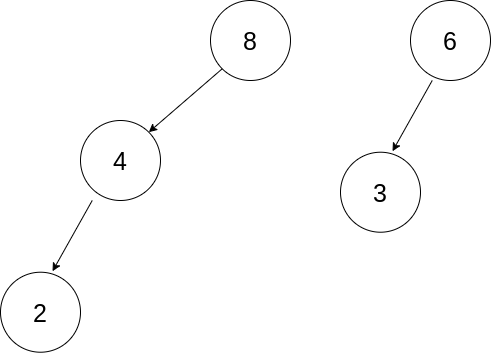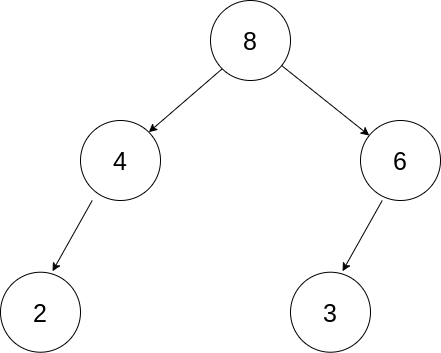# Minimize Cost for Reducing Array by Replacing Two Elements with Sum at most K times for any Index

## Understanding

This blog discusses a coding problem based on n-ary trees and prefix sums. The blog will present a challenge that involves tricky observations and constructions. The problem will challenge you to reimagine the problem differently to get to the solution. Such problems are increasingly asked in programming contests where you need to transform a question to relate it with a different concept where you can construct things that resemble entities in the original problem.

## Problem Statement

Ninja has given you an array, ‘ARR’ and an integer ‘K’. Your task is to determine the minimum possible cost to collect the sum of the array. You can collect the sum of the array elements by using the given operation as many times as possible till the restriction proposed is true:

1. Pick two indices, ‘INDEX1’ and ‘INDEX2’, and add ‘ARR[INDEX2]’ to ‘ARR[INDEX1]’.
2. Cost of collection += ARR[INDEX2].
3. Make ARR[INDEX2] = 0.

Restriction: The addition of elements at the same index is allowed at most K times. It means for any valid index, ‘INDEX’, you can add elements of other indices to this index at most ‘K’ times.

### Input

ARR: {4, 8, 6, 2, 3}

K = 2

20

### Explanation

You can do the following operations:

1. Add ‘ARR’ to ‘ARR’. ARR = {6, 8, 6, 0, 3}
2. Add ‘ARR’ to ‘ARR’. ARR = {6, 8, 9, 0, 0}
3. Add ‘ARR’ to ‘ARR’. ARR = {0, 14, 9, 0, 3}
4. Add ‘ARR’ to ‘ARR’. ARR = {0, 23, 0, 0, 0}

We have added elements of other indices to index 1 two times, which is within limits.

## Approach

To solve this problem, we need to transform this problem into a graphical one. Let's first sort the array in non-increasing order. Now, let's assume each array element represents a node in a k-ary tree. A k-ary tree is a tree where each node has at most ‘K’ children.

We can visualise the operation of adding an array element, ‘ELEM1’ to another element ‘ELEM2’ as appending the subtree of ‘ELEM1’ as a child to ‘ELEM2’. We can append the subtree of ‘ELEM1’ to ‘ELEM2’ only if ‘ELEM2’ has fewer than ‘K’ children.

Now, as we have identified an operation in trees that resemble the addition operation described in the problem, let's move on to the definition of cost in the new context.

The cost of collecting the sum of an array can be calculated by summing up the multiplication of each node with its depth in the tree, assuming the root is at depth 0.

To understand this, let's take the example mentioned before.

Let's create the tree corresponding to the operations. Each previously mentioned operation translates to an edge as shown in the images below.

The initial configurationOperation 1Operation 2Operation 3Operation 4Cost = 8 * 0 + 4 * 1 + 6 * 1 + 2 * 2 + 3 * 2 = 20

Now, the question is how to minimize the cost?

Taking intuition from the tree structure, we can do the following:

1. Nodes with higher values should have lower depth.
2. Each non-leaf node should have K children to accumulate as many nodes as possible in the lower depths.

Remember, we need not create the tree. We just did this analysis to understand the problem better.

### Algorithm

1. Take the input array and integer K.
2. Sort the array in non-increasing order.
3. Calculate the prefix sum in the ‘PREF_SUM’ array.
4. Initialise the following variables:
1. COST: To store the cost of collection.
2. START_INDEX: It will depict the index of the leftmost node at each level.
3. DEPTH: To store the current depth as the algorithm runs.
4. DEPTH_SIZE: To hold the number of nodes at each depth.
5. While the ‘START_INDEX’ is less than ‘N’, do the following:
1. Calculate END_INDEX = START_INDEX + DEPTH_SIZE.
2. Take the minimum of ‘END_INDEX’ and ‘N - 1’ and store it in ‘END_INDEX’
3. Calculate the sum of elements in the range described by ‘START_INDEX’ and ‘END_INDEX’ using ‘PREF_SUM’ array.
4. Increment ‘COST’ by the sum calculated in previous step multiplied by the current depth.
5. Increment ‘DEPTH’ by one.
6. DEPTH_SIZE *= K to take into account the second point on minimising the cost.
6. Output the COST.

### Program

``````#include<iostream>
#include<algorithm>
using namespace std;

int findCost(int arr[])
{
// Size of the array.
int N = sizeof(arr)/sizeof(arr);

// Integer K as described in the problem statement.
int K = 2;

// Sort the array in the non-increasing order.
sort(arr, arr + N, greater<int>());

// Prefix sum calculation.
int prefSum[N+1];
prefSum = 0;

// Standard technique to compute prefix sum in O(N) time.
for(int i = 0; i < N; i++)
{
prefSum[i + 1] = prefSum[i] + arr[i];
}

// Cost of sum collection.
int cost = 0;

// Elements to pick from. 0th index is ignored as it will always be multiplied by zero, thus contribution to the cost.
int startIndex = 1;

// Current depth.
int depth = 1;

// Number of nodes at the current depth.
int depthSize = K;
while(startIndex < N)
{
// Array elements covered at this depth.
int endIndex = startIndex + depthSize - 1;

// In case of overflow.
endIndex = min(endIndex, N - 1);

// Sum of elements at the current depth.
int levelSum = prefSum[endIndex + 1] - prefSum[startIndex];

// As described by the approach.
cost += levelSum * depth;

// Update the startIndex after covering all the elements till endIndex.
startIndex = endIndex + 1;

// The number of nodes at the next level will be K times the number of nodes at this level.
// This is to accumulate as many nodes as possible in the lower depths.
// Level is used interchangeably with depth.

depthSize *= K;
// Increase the depth.
depth++;
}
}
int main()
{
int N;
cout << "Enter the size of the array: ";
cin >> N;

// Input array.
int arr[N];
cout << "Enter the elements: ";
for(int i = 0; i < N; i++){
cin >> arr[i];
}

cout << findCost(arr) << endl;
}``````

### Input

``````Enter the size of the array: 5
Enter the elements: 4 8 6 2 3``````

### Output

``20``

### Time Complexity

The time complexity of the above approach is O(N * logN), where N is the size of the input array.

It is because we are sorting the array in non-increasing order which takes O(N * log N). The time complexity of the while loop is O(logKN) where K is the input parameter, however, the sorting algorithm is the upper bound to the time complexity.

### Space Complexity

The space complexity of the above approach is O(N) to store the prefix sum array.

## Key Takeaways

This blog discussed a very interesting problem based on prefix sums and k-ary trees. It was intriguing to analyse the problem graphically. We created operations in the graphical domain that resemble operations described in the problem. The most important part and the motive of the construction was to understand how to minimise the cost of collecting the sum.

Hence learning never stops, and there is a lot more to learn.

So head over to our practice platform CodeStudio to practice top problems, attempt mock tests, read interview experiences, and much more. Till then, Happy Coding!